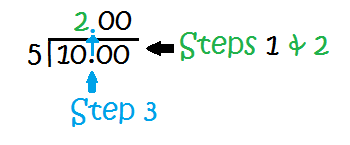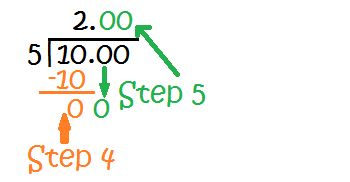# Dividing Decimals by Whole Numbers

Instructor: Emily Hume

Emily is a Reading Specialist and Literacy coach in a public elementary school with a Master's Degree in Elementary Education.

Have you ever had to divide a decimal by a whole number? Bet you did and didn't even know it! If you've used money, you've used decimals. This lesson will teach you how to divide a decimal by a whole number!

## Where Are Decimals Used?

Hooray - you earned \$10.00 by raking leaves! Mom says you must save it for your vacation next week. If your vacation is five days long, how much can you spend each day?

You could get change and divide it up among each day. This would take awhile and be lots of work! There is a better way to figure this out. You can use division!

## Steps to Divide Decimals by Whole Numbers

Before solving, you have to know what decimals and whole numbers are. Decimals have a period (called a decimal point) in them. A decimal is a fraction, or a part, of a number. For example, .50 is a decimal because it is less than one. A whole number is a positive number, including zero, that is neither a fraction nor a decimal.

Let's solve your spending money problem!

Step 1: Set up your problem. 10.00 ÷ 5. Another way to write it is: Write the 5, then a straight line (|), followed by 10.00 with a line ¯¯¯ above it.

Step 2: Find the first number that 5 will go into. Does it go into 1? Nope! How about 10? Yes! 5 goes in to 10 two times (10 ÷ 5 = 2). Write '2' above the '0' in 10.

Step 3: Move the decimal point straight up so that it becomes part of your answer. In this case, it will come after the '2' in the answer.

See the image: Dividing Decimals by Whole Numbers Steps 1-3, for a visual of Steps 1-3!Step 4: Multiply the number on the outside by the number on the top (in this case, 5 x 2), put the 10 under the number you just divided into (in this case, 10) and then subtract.

Step 5: Bring the next number down. In this case, 0. Since the rest of the numbers in 10.00 are zero, you can fill in the zeroes without having to bring them down and divide.

See the image: Dividing Decimals Steps 4 and 5 for a visual on these steps!Looks like you can spend \$2.00 per day on your 5-day vacation! Awesome!

## Another Example of Dividing a Decimal

Decimals don't always refer to money. Let's try another example!

To unlock this lesson you must be a Study.com Member.

### Register to view this lesson

Are you a student or a teacher?